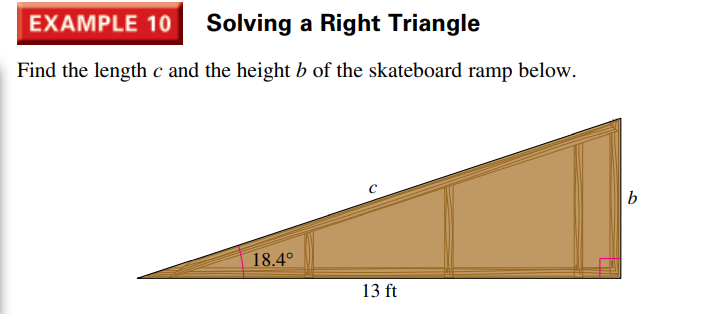### Still have math questions?

Trigonometry
QuestionEXAMPLE $$10$$ Solving a Right Triangle Find the length $$c$$ and the height $$b$$ of the skateboard ramp below.